# Fluid Mechanics - Fluid Properties

18. Oct 2020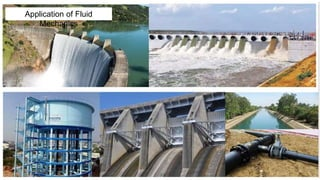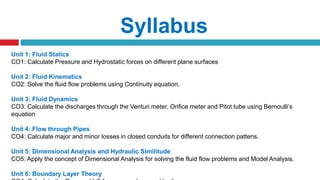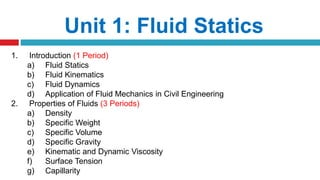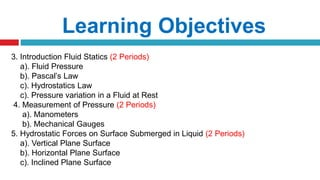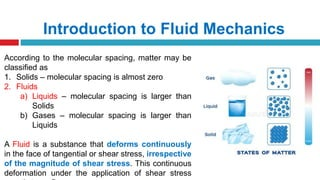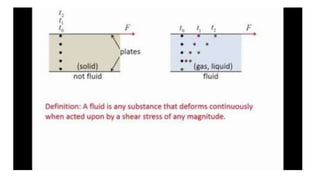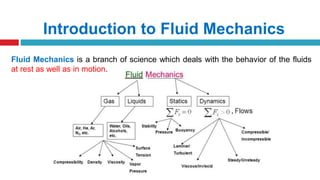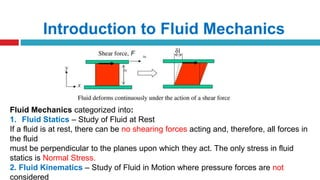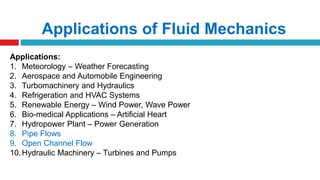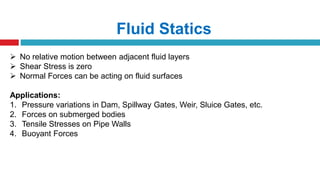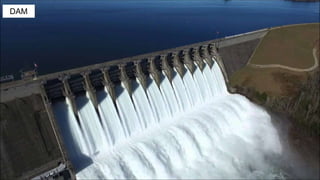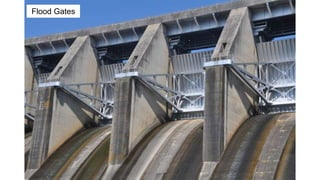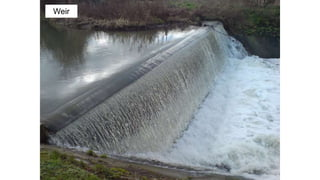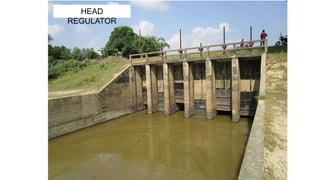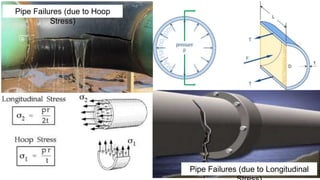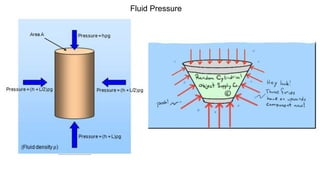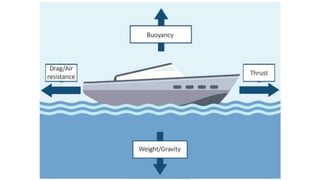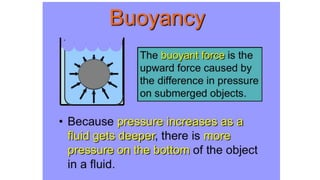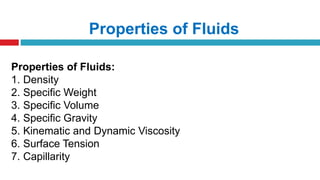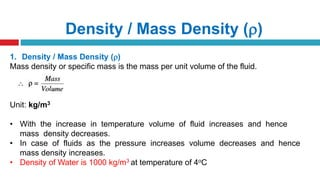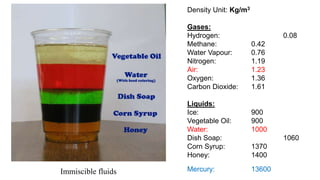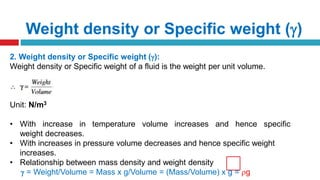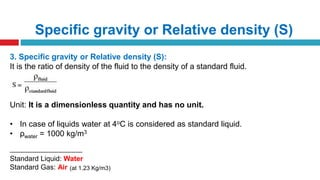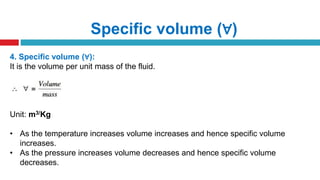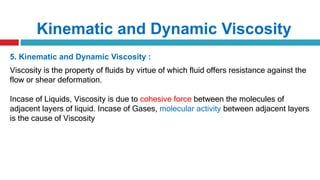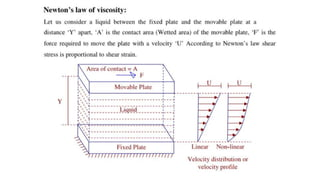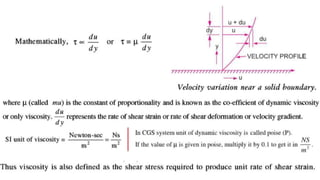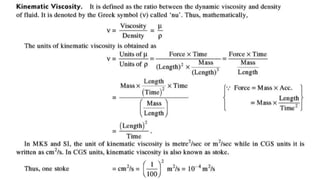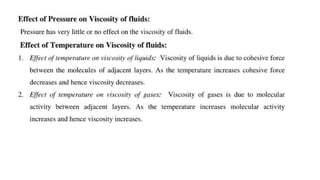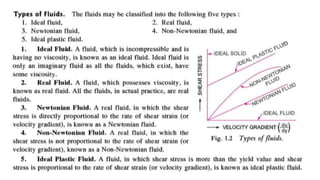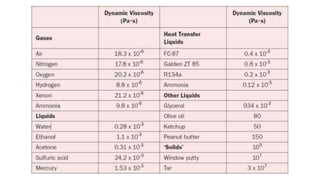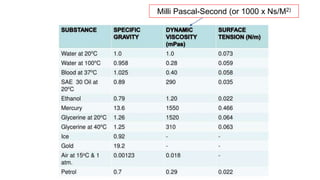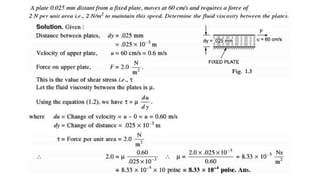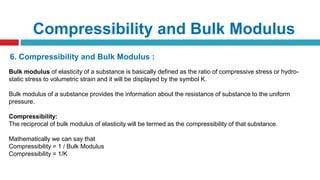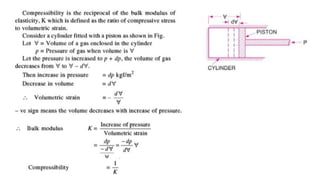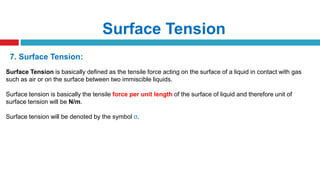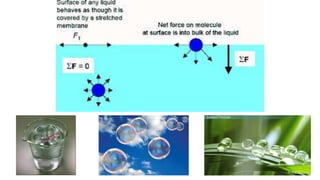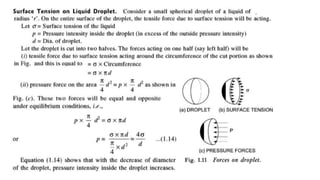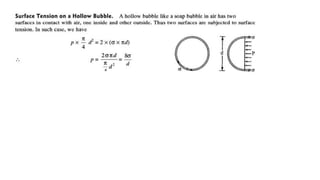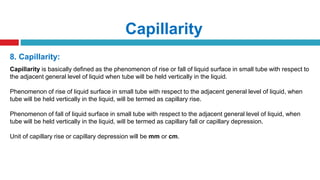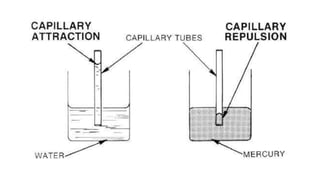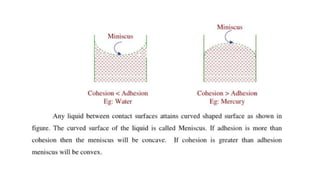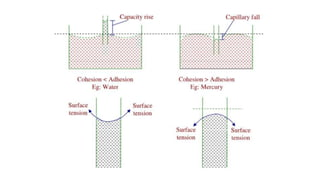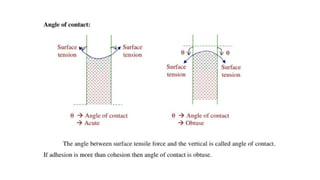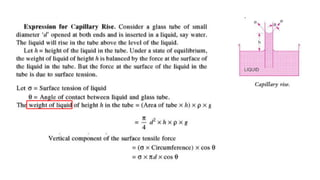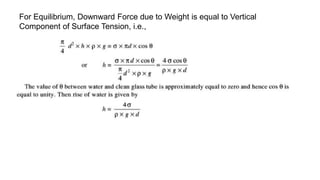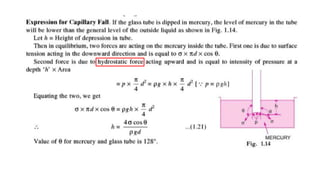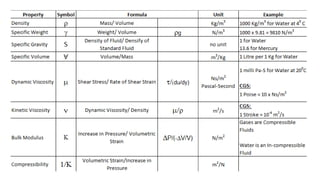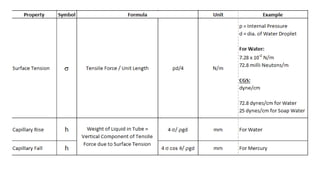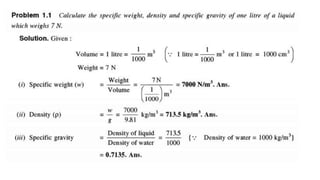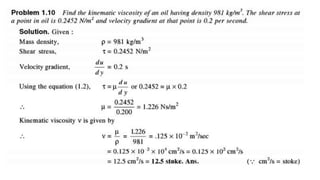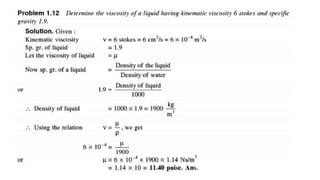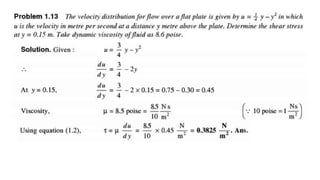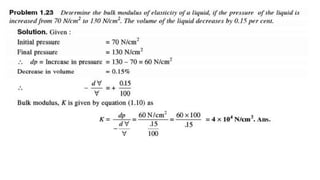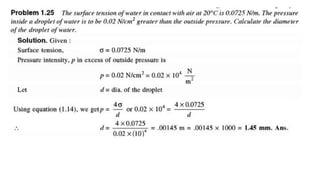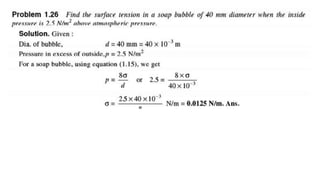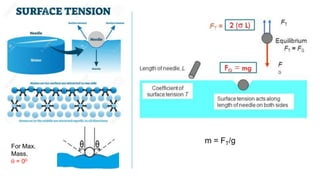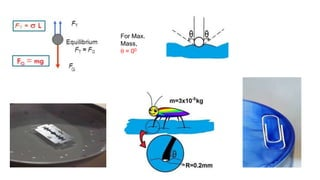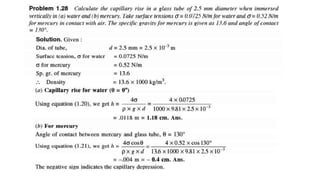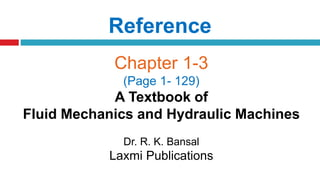1 von 61

### Fluid Mechanics - Fluid Properties

• 1. FLUID PROPERTIES (FLUID MECHANICS) UNIT – I Dr. Rambabu Palaka, ProfessorJuly-2020
• 3. Syllabus Unit 1: Fluid Statics CO1: Calculate Pressure and Hydrostatic forces on different plane surfaces Unit 2: Fluid Kinematics CO2: Solve the fluid flow problems using Continuity equation. Unit 3: Fluid Dynamics CO3: Calculate the discharges through the Venturi meter, Orifice meter and Pitot tube using Bernoulli’s equation Unit 4: Flow through Pipes CO4: Calculate major and minor losses in closed conduits for different connection pattens. Unit 5: Dimensional Analysis and Hydraulic Similitude CO5: Apply the concept of Dimensional Analysis for solving the fluid flow problems and Model Analysis. Unit 6: Boundary Layer Theory
• 4. Unit 1: Fluid Statics 1. Introduction (1 Period) a) Fluid Statics b) Fluid Kinematics c) Fluid Dynamics d) Application of Fluid Mechanics in Civil Engineering 2. Properties of Fluids (3 Periods) a) Density b) Specific Weight c) Specific Volume d) Specific Gravity e) Kinematic and Dynamic Viscosity f) Surface Tension g) Capillarity
• 5. Learning Objectives 3. Introduction Fluid Statics (2 Periods) a). Fluid Pressure b). Pascal’s Law c). Hydrostatics Law c). Pressure variation in a Fluid at Rest 4. Measurement of Pressure (2 Periods) a). Manometers b). Mechanical Gauges 5. Hydrostatic Forces on Surface Submerged in Liquid (2 Periods) a). Vertical Plane Surface b). Horizontal Plane Surface c). Inclined Plane Surface
• 6. Introduction to Fluid Mechanics According to the molecular spacing, matter may be classified as 1. Solids – molecular spacing is almost zero 2. Fluids a) Liquids – molecular spacing is larger than Solids b) Gases – molecular spacing is larger than Liquids A Fluid is a substance that deforms continuously in the face of tangential or shear stress, irrespective of the magnitude of shear stress. This continuous deformation under the application of shear stress
• 8. Introduction to Fluid Mechanics Fluid Mechanics is a branch of science which deals with the behavior of the fluids at rest as well as in motion.
• 9. Introduction to Fluid Mechanics Fluid Mechanics categorized into: 1. Fluid Statics – Study of Fluid at Rest If a fluid is at rest, there can be no shearing forces acting and, therefore, all forces in the fluid must be perpendicular to the planes upon which they act. The only stress in fluid statics is Normal Stress. 2. Fluid Kinematics – Study of Fluid in Motion where pressure forces are not considered
• 10. Applications of Fluid Mechanics Applications: 1. Meteorology – Weather Forecasting 2. Aerospace and Automobile Engineering 3. Turbomachinery and Hydraulics 4. Refrigeration and HVAC Systems 5. Renewable Energy – Wind Power, Wave Power 6. Bio-medical Applications – Artificial Heart 7. Hydropower Plant – Power Generation 8. Pipe Flows 9. Open Channel Flow 10.Hydraulic Machinery – Turbines and Pumps
• 11. Fluid Statics  No relative motion between adjacent fluid layers  Shear Stress is zero  Normal Forces can be acting on fluid surfaces Applications: 1. Pressure variations in Dam, Spillway Gates, Weir, Sluice Gates, etc. 2. Forces on submerged bodies 3. Tensile Stresses on Pipe Walls 4. Buoyant Forces
• 12. DAM
• 14. Weir
• 16. Pipe Failures (due to Hoop Stress) Pipe Failures (due to Longitudinal
• 20. Properties of Fluids Properties of Fluids: 1. Density 2. Specific Weight 3. Specific Volume 4. Specific Gravity 5. Kinematic and Dynamic Viscosity 6. Surface Tension 7. Capillarity
• 21. Density / Mass Density () 1. Density / Mass Density () Mass density or specific mass is the mass per unit volume of the fluid. Unit: kg/m3 • With the increase in temperature volume of fluid increases and hence mass density decreases. • In case of fluids as the pressure increases volume decreases and hence mass density increases. • Density of Water is 1000 kg/m3 at temperature of 4oC
• 22. Density Unit: Kg/m3 Gases: Hydrogen: 0.08 Methane: 0.42 Water Vapour: 0.76 Nitrogen: 1.19 Air: 1.23 Oxygen: 1.36 Carbon Dioxide: 1.61 Liquids: Ice: 900 Vegetable Oil: 900 Water: 1000 Dish Soap: 1060 Corn Syrup: 1370 Honey: 1400 Mercury: 13600Immiscible fluids
• 23. Weight density or Specific weight () 2. Weight density or Specific weight (): Weight density or Specific weight of a fluid is the weight per unit volume. Unit: N/m3 • With increase in temperature volume increases and hence specific weight decreases. • With increases in pressure volume decreases and hence specific weight increases. • Relationship between mass density and weight density  = Weight/Volume = Mass x g/Volume = (Mass/Volume) x g = g
• 24. Specific gravity or Relative density (S) 3. Specific gravity or Relative density (S): It is the ratio of density of the fluid to the density of a standard fluid. Unit: It is a dimensionless quantity and has no unit. • In case of liquids water at 4oC is considered as standard liquid. • ρwater = 1000 kg/m3 ----------------------------------------- Standard Liquid: Water Standard Gas: Air (at 1.23 Kg/m3)
• 25. Specific volume (∀) 4. Specific volume (∀): It is the volume per unit mass of the fluid. Unit: m3/Kg • As the temperature increases volume increases and hence specific volume increases. • As the pressure increases volume decreases and hence specific volume decreases.
• 26. Kinematic and Dynamic Viscosity 5. Kinematic and Dynamic Viscosity : Viscosity is the property of fluids by virtue of which fluid offers resistance against the flow or shear deformation. Incase of Liquids, Viscosity is due to cohesive force between the molecules of adjacent layers of liquid. Incase of Gases, molecular activity between adjacent layers is the cause of Viscosity
• 33. Milli Pascal-Second (or 1000 x Ns/M2)
• 35. Compressibility and Bulk Modulus 6. Compressibility and Bulk Modulus : Bulk modulus of elasticity of a substance is basically defined as the ratio of compressive stress or hydro- static stress to volumetric strain and it will be displayed by the symbol K. Bulk modulus of a substance provides the information about the resistance of substance to the uniform pressure. Compressibility: The reciprocal of bulk modulus of elasticity will be termed as the compressibility of that substance. Mathematically we can say that Compressibility = 1 / Bulk Modulus Compressibility = 1/K
• 37. Surface Tension 7. Surface Tension: Surface Tension is basically defined as the tensile force acting on the surface of a liquid in contact with gas such as air or on the surface between two immiscible liquids. Surface tension is basically the tensile force per unit length of the surface of liquid and therefore unit of surface tension will be N/m. Surface tension will be denoted by the symbol σ.
• 41. Capillarity 8. Capillarity: Capillarity is basically defined as the phenomenon of rise or fall of liquid surface in small tube with respect to the adjacent general level of liquid when tube will be held vertically in the liquid. Phenomenon of rise of liquid surface in small tube with respect to the adjacent general level of liquid, when tube will be held vertically in the liquid, will be termed as capillary rise. Phenomenon of fall of liquid surface in small tube with respect to the adjacent general level of liquid, when tube will be held vertically in the liquid, will be termed as capillary fall or capillary depression. Unit of capillary rise or capillary depression will be mm or cm.
• 47. For Equilibrium, Downward Force due to Weight is equal to Vertical Component of Surface Tension, i.e.,
• 58. For Max. Mass,  = 00 m = FT/g
• 61. Reference Chapter 1-3 (Page 1- 129) A Textbook of Fluid Mechanics and Hydraulic Machines Dr. R. K. Bansal Laxmi Publications